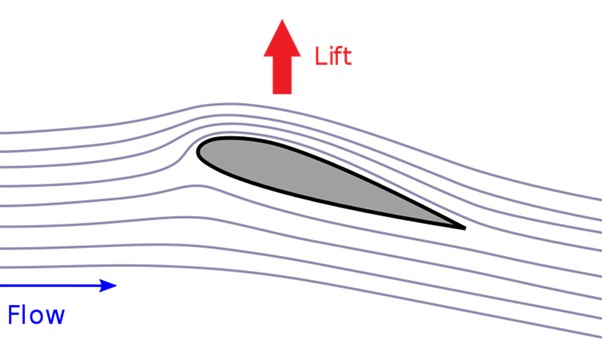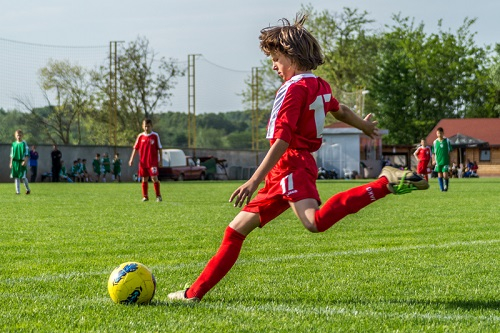# Dynamic Lift

## Introduction

The particle is considered to be in motion when it changes its position to time. That means the particle is displaced from one position to another. The particle makes linear displacement If it is moving in linear motion. Similarly, the particle makes angular displacement if it is moving in a circular motion. That means circularly moving particles make angular displacement. There are laws describing the motion of particles. According to the first law, the particle does not change its state of motion or rest without any external force. The second law indicates that the force acting on the particle depends upon the mass of the particle and acceleration of the particle.

$$\mathrm{F=ma}$$

Then the third law shows that if a force is applied to the particle then there should be an equal force produced in the opposite direction. Let us see about the spinning movement of objects. The subset of matters such as liquid, gas, and solid is fluid. Fluid is a liquid, gas, or other material that can deform due to the external force acting on it.

## What is a Dynamic Lift?

If an object is moving through a fluid it experiences a force due to the motion of the object. This force is called dynamic lift. Let us consider an airplane that flies in the air. The air under the wing of the airplane exerts an upward force on the plane known as dynamic lift.

## Principle of Dynamic Lift

According to Bernoulli’s principle, the velocity of the upper layer is greater than the velocity of the lower layer. Thus the pressure of the lower layer is greater than the upper layer. This pressure difference generates a force that lifts the object. Bernoulli’s theorem is the basic principle of dynamic lifting. There is a decrease in potential energy or a decrease in static pressure with an increase in the speed of the fluid. This is the principle of dynamic lift.

## Basic terms related to Dynamic Lift

The dynamic lift that happens as a result of spinning is called the Magnus effect. This phenomenon is based on the spinning movement of an object through the liquid. Here the path of the spinning object is deflected from its path. It does not happen if the object is not spinning. It is explained by the pressure difference of the fluid. Magnus effect of the spinning object depends upon the speed of the spinning object. Let us consider an object that moves from right to left with clockwise spinning, then top layered particles get accelerated and low lying particles get slowed down by the force. The Magnus effect and Bernoulli's theorem are interrelated with each other.

## Working on the Dynamic Lift

For non-spinning balls, the flow of fluid around the ball is in streamlined motion. In a streamlined motion, the velocity of fluid above and below the ball at a particular point is the same so there is no pressure difference between the layer above and below the ball. Thus there is no upward and downward force acting on the ball.Michael Paetzold, Lizenz: Creative Commons by-sa-4.0, DeflectionAndLift W3C, CC BY-SA 4.0

For a spinning ball, the air is dragged towards the motion of the ball. If the ball moves in the forward direction the air movement is in the backward direction. Thus there occurs a difference in relative velocity. As there is a difference in velocity of the air above and below the ball there occurs a pressure difference between them. There occurs a net upward force because the velocity is higher for air above than below. Due to this, the ball experiences an upward lift called the Magnus effect.

## Examples of Examples of Dynamic Lift

• The dynamic lift has applications in many fields such as aerodynamics and sports. In the football game, the player tends to change the direction of the ball to the left side. By kicking it with the right leg the spin of the ball is in an anticlockwise direction. As the air on the left side of the ball is in the direction of the motion of the ball it gets accelerated. The air on the right side of the ball is opposite to the direction of motion of the ball. So air on the right side gets decelerated. Thus there occurs a velocity gradient on the two sides of the ball which in turn the pressure on the left and right sides of the ball becomes low and high. Due to this pressure difference, the ball is pushed toward the lower pressure side. That is, the ball turns to the left side. This is how the Magnus effect is applied.• In airplanes, four forces make them fly. Lift force makes the flight lift. The pressure difference in the air around the wing exerts a force that lifts the flight. Airplanes are manufactured as the weight of the flight is evenly spread from front to back. As long as the air goes through the wing engine gives thrust to the flight which makes it move forward. Drag force that slows down the flight. They are designed to let the air pass around to have less drag force.

## Conclusion

If the particle changes its position means it is in motion. The motion of an object is explained by three laws proposed by Newton. Dynamic drift is an important phenomenon in fluid dynamics. That was explained in this article. Beyond that, the principle of dynamic lift and basic terms that are related to dynamic lift is also discussed here. The examples that best explain dynamic lift are also discussed.

## FAQs

Q1. What are the forces that are needed for a flight to fly?

Ans. Four forces are needed for an airplane to fly. They are dynamic lift, gravity, thrust, and drag. Dynamic lift is a force that acts on the flight for upward action. The weight of the flight acts as a downward action. Thrust and drag are the forces that lead the flight in forwarding and backward directions.

Q2. State Bernoulli’s theorem.

Ans. It states that when the flow of liquid is steady, the total energy of the system remains constant. When the speed of the fluid increases then the pressure of the fluid decreases. According to this for incompressible liquid, the sum of potential energy, kinetic energy, and the pressure energy of the fluid remains constant.

$$\mathrm{P+1/2\rho v^{2}+\rho gh=constant}$$

P denotes the pressure inside the fluid. ρ denotes the density of the fluid

Q3. What is aerodynamic force?

Ans. When a body is immersed in the air or gas, a force acts on the body due to the relative motion that occurs between the body and the gas. That force is called aerodynamic force. Two resolved components of this aerodynamic force are drag and lift. In this drag, a force acts parallel to the direction of relative motion and the lift force acts perpendicular to the direction of relative motion.

Q4. What are the applications of the Magnus effect?

Ans.

• Magnus effect is used in many sports like football, golf, cricket, tennis, etc.

• Some aircraft use the Magnus effect.

• In rotor ships also this concept was used.

• It is applicable in ballistics.

Q5. What are the limitations of Bernoulli’s theorem?

Ans.

• Bernoulli’s theorem is applied only for the fluid which is in streamlined motion. And it is not applicable for turbulent or non-steady flow.

• The flow of liquid will be affected by the external force.

• Viscous liquids are not suitable for this theorem.

• The most important criterion is that the fluid must be incompressible.

• The flow of liquid must be constant in time.

• If the flow of fluid is in a circular path then the energy due to centrifugal force must be taken into consideration.

These are all the limitations of Bernoulli’s theorem.

Updated on: 24-Jan-2023

227 Views